# ISEE Lower Level Math : How to identify the parts of a list

## Example Questions

← Previous 1 3 4 5

### Example Question #1 : How To Identify The Parts Of A List

The data below represent the ages of the people attending the Morton family reunion. Find the range for the data.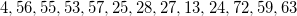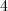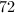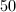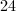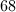Explanation:

In order to find the range, we must find the difference between the largest number in the data and the smallest number in the data. The easier way to locate the smallest and largest numbers in the data is to put them in order of smallest to largest.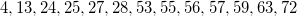By doing this we can see that 72 is our largest number and 4 is our smallest number. Now we find the difference between these two numbers using subtraction.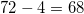### Example Question #1 : How To Identify The Parts Of A List

These are the test scores on the final exam for Ms. Robinson’s math class: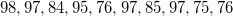. What is the mode of these scores?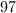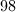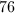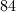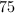Explanation:

In order to find the mode of a set of data, we must find the number that occurs the most often. In this set,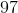appears three times, making it the most common number in the data set, so it is the mode.

### Example Question #3 : How To Identify The Parts Of A List

In Mr. Shoemaker’s class, the students took a poll to see how many siblings each student in the class had. Five of the students reported having 1 sibling. Two students reported having no siblings. Three reported having 4 siblings. Seven reported having 3 siblings. This data can be represented as: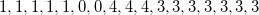. What is the median of this data.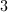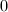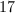Explanation:

The median of a set of data is the number that is physically in the middle of that data set when it is displayed in order from smallest to largest. In order to find the median of this data, we must first put it in order of smallest to largest.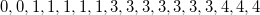Next we determine how many students there are by adding together how many students reported having each number of siblings.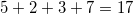Since seventeen is an odd number, one number will represent the median, and that number will be the 9th number in the sequence. We know this because the 9th number has eight on either side of it, putting it exactly in the middle of the data.

In our sequence, the 9th number is, making the median of our data.

### Example Question #4 : How To Identify The Parts Of A List

Maribel bought a pack of colorful rubber bands to make bracelets for her friends.  In the pack of rubber bands there were 17 yellow, 3 black, 21 orange, 13 red, 40 blue and 6 green.  Which color would be the mode of the pack?

Blue

Yellow

Orange

Red

Black

Blue

Explanation:

The mode is defined as the MOST in appearance. In the pack of rubber bands the color blue would appear the most in the pack.

Therefore, the mode [MOST] is Blue.

### Example Question #5 : How To Identify The Parts Of A List

Define the setWhich of the following is not a subset of?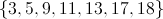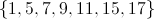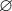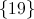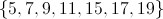Explanation:

A subset of a set, by definition, is any set that contains no elements not in. All elements in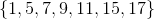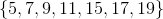, and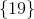are contained inand the sets are subsets; also,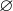, the empty set, is considered a subset of every set.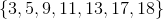, however, contains element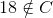, so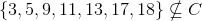.

### Example Question #6 : How To Identify The Parts Of A List

Define two sets as follows: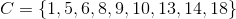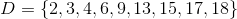How many elements are in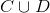?Explanation:is the union of setsand- the set of all elements that appear in eitheror. These elements are: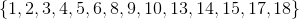This is a set of 14 elements.

### Example Question #7 : How To Identify The Parts Of A List

Define a setas follows:Which of the following is a subset of?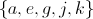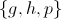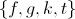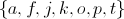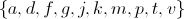Explanation:

A subset of a set, by definition, is any set that contains no elements not in.  Each of the following subsets can be seen to have at least one such element, which is underlined here: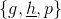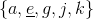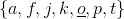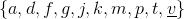The remaining set can be seen to have only elements from: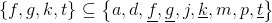This is the correct choice.

### Example Question #8 : How To Identify The Parts Of A List

Ms. Howard gave the students a test last Friday and they are reviewing it in class today.

The student scores were as follows:

Carla   80

Ben     75

Joe      90

Paul    100

Zoe     70

What was the median score for the test?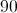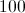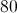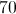Explanation:

To find the median the set of numbers must be put in order [least to greatest OR greatest to least].  Once the numbers are in order, then cross out one number from each side of the list until the middle of the ordered numbers is reached.

The number in the middle of the ordered numbers is the median.

Placing the scores in order from least to greatest the list would appear as follows:

70, 75, 80, 90, 100

Cross out one number at a time from each side.

First cross out 70. Next, cross out 100.  Then, cross out 75. Finally, cross out 90.

70, 75, 80, 90, 100

The median is the number left in the middle of the ordered list which is 80.

### Example Question #9 : How To Identify The Parts Of A List

What is the mode of the following set of numbers?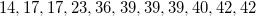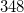Explanation:

The mode is the number that is repeated most often.is repeated three times, more than any other number in the set.

### Example Question #10 : How To Identify The Parts Of A List

What is the mode of the following set of numbers?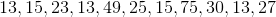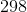The mode of a set of numbers is the number that is repeated most often. In this example,is repeated three times, more than any other number in the set. Therefore,is the mode.